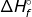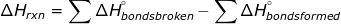# Enthalpy Formula

Enthalpy Formula

Enthalpy is a thermodynamic function that is equal to the total internal energy of the system plus the product of pressure and volume. The equation is as follows:

H = E + PV

where H is the enthalpy, E is the energy and PV is the pressure multiplied by the volume.

The total internal energy of a system is impossible to calculate, but changes in internal energy can. The changes involve heat transfer and work done (the expansion or contraction of a gas). Therefore the enthalpy of a reaction is noted as ΔH where the symbol Δ refers to the change. The ΔH of the reaction () can be calculated in many different ways.

1. If the work done by or on a system is zero (the volume of the container does not change) then the change in enthalpy is equal to the heat transfer (q), where. In this equation m is the mass, s is the specific heat, and ΔT is the change in temperature.

2. If the reaction is known, a table ofvalues can be used to calculate the. Theis called the heat of formation, and it refers to the heat is takes to form the substance from its elements.3. Hess's Law could be used to calculate the enthalpy of a reaction.

4. Thecan be calculated by using the bond energies of the reactants and products.Enthalpy Formula Questions:1. Calculate the heat of the following reaction using the table ofvalues.

C(s) + O2 (g) → CO2 (g)Theis called the heat of formation, and it refers to the heat is takes to form the substance from its elements. Thefor C(s) and O2 (g) have values of 0 because they are in elemental form.= (-393.5)-(0 + 0) = -393.5kj2. Calculate the heat of the following reaction using the table ofvalues.

CH4 (g) + 2O2 (g) → CO2 (g) + 2H2O(l)In this problem there are 2 moles of O2 and 2 moles of H2O so theirvalues must be multiplied by two.= [-393.5 + (2*285.8)] - [-74.80 + (2*0)] = -890.3kj